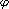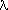# Brunt Vaisala or Buoyancy Frequency

N2 = (Rg/H(z)) · [ dT0/dz + (Rg/Cp) · (T0(z)/H(z)) ] = (Rg/H(z)) · [ dT0/dz + g/Cp ] = (Rg/H(z)) ·where, T0(z) = <T(z,,,t)>, i.e., temperature averaged over longitude, latitude, and time t.
The static stability,, is related to the buoyancy frequency asdT0/dz + g/Cp
The static stability is positive for stable conditions and zero or negative for unstable conditions.